## 3.5.1 Response Distance of an Ambulette, Revisited

As in Example I of Section 3. 1, suppose that X1 and X2 are independent and uniformly distributed over the interval [0, a]. We want the mean travel distance, which we call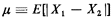Clearly, this expected value can be obtained easily by our earlier (direct) methods, but this simple example will illustrate the basic idea of Crofton's method.

We add to the interval [0, a] an increment of length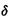a, as shown in Figure 3.27. We now consider the problem in which X1 and X2 are independent and distributed in the same way as before, but over the larger interval [0, a +a]. Let the mean of |X1 - X2| over [0, a +a] be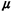+. Consider the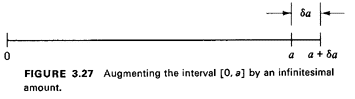following four mutually exclusive events:The key to Crofton's method is that it isolates one of the points in the infinitesimal interval, thereby yielding a quantity1, which is the mean value of the random variable, given that one of the points is located in the infinitesimal interval. This quantity is usually easier to compute than(since one of the points is "pinned down").

In this problem it is obvious that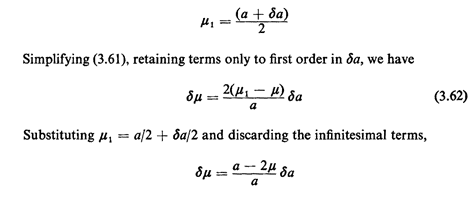which yields the differential equation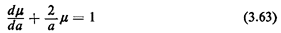As with most differential equations, this one has a homogeneous and a particular solution. The homogeneous solution is= Kh/a2 for some constant K, Here K, must be set equal to zero, since otherwisebecomes infinitely large as a approaches zero, a result obviously not physically possible (since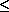a). Motivated by physical considerations (and the result of scaling random variables-Section 3.1), we propose as a particular solution= Kpa. Substituting in (3.63), we find K, thus corroborating our earlier result of Example 1.

Examining the derivation, we could retain1 symbolically in the differential equation implied by (3.62), yielding6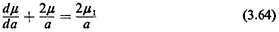Note that this differential equation has been derived considering only the geometry of the region R and the uniform probability laws of X1 and X2; in particular, the specific form of the function whose expected value is1 has not been considered in the derivation.

For example, if we identifywith the second moment, that is,=E[| X1 - X2|1], then clearlyCombining our two results, we find for the variance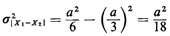In general, Crofton's method can be used to find any of the moments of the random variable of interest.

In many cases we can extend these ideas to find the probability distribution of the random variable. We do this by invoking a set indicator random variable. A random variable XA is said to be an indicator random variable for the set A if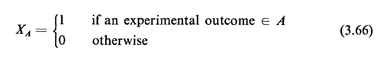The utility of the set-indicator random variable derives from its simplicity (only two possible values) and the fact that
E[XA] = 0·(I - PfA}) + I ·P{A = P {A}
In other words, the expected value of the set-indicator random variable is equal to the probability that an outcome of the corresponding experiment is contained in the set indicated.

In our continuing example, suppose that we define the set-indicator random variable as follows: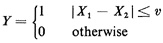Now the expected value of Y will equal the probability that |X1 - X2|D is less than or equal to v; thus, in this case,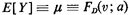This is the essential relationship one requires to apply Crofton's ideas to deriving probability laws of functions of random variables (very special random variables, to be sure). Hence, solving Crofton's problem in this instance provides us with the cdf for the random variable |X1 - X1| = D. HereBut this is equivalent to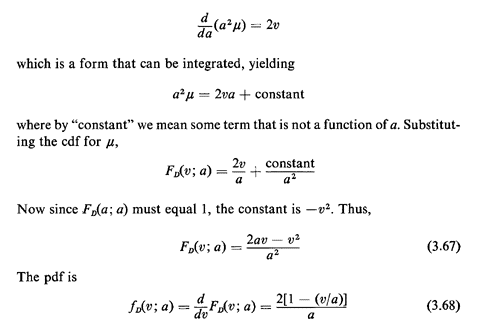which confirms earlier results (Example 1).

Crofton's method can be generalized to situations in which there are N points distributed independently and uniformly over R.

Exercise 3.6: Crofton's Method Applied to N Points Suppose that N points are distributed uniformly and independently over [0, a). Letbe the expected value of some function of the relative positions of the points. Let1 be the expected value of the same function, conditioned on the event that exactly one of the points is "pinned down" in the infinitesimal interval [a, a +a]. Show that6Technically, given the definition of1 in (3.61),1 on the right hand side of (3.64) should be replaced with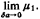. For simplicity of notation, we have chosen to ignore the infinitesimal term involved since it plays no role in the resulting differential equation.